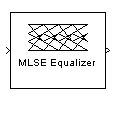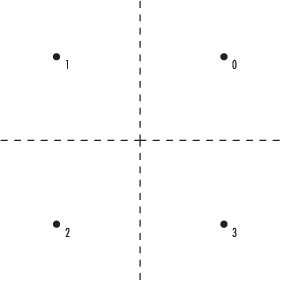Main Content

# MLSE Equalizer

Equalize using Viterbi algorithm

## Library

Equalizer Block

•## Description

The MLSE Equalizer block uses the Viterbi algorithm to equalize a linearly modulated signal through a dispersive channel. The block processes input frames and outputs the maximum likelihood sequence estimate (MLSE) of the signal, using an estimate of the channel modeled as a finite input response (FIR) filter.

This block supports `single` and `double` data types.

### Channel Estimates

The channel estimate takes the form of a column vector containing the coefficients of an FIR filter in descending order of powers. The length of this vector is the channel memory, which must be a multiple of the block's Samples per input symbol parameter.

To specify the channel estimate vector, use one of these methods:

• Set Specify channel via to `Dialog` and enter the vector in the Channel coefficients field.

• Set Specify channel via to ```Input port``` and the block displays an additional input port, labeled `Ch`, which accepts a column vector input signal.

### Signal Constellation

The Signal constellation parameter specifies the constellation for the modulated signal, as determined by the modulator in your model. Signal constellation is a vector of complex numbers, where the kth complex number in the vector is the constellation point to which the modulator maps the integer k-1.

Note

The sequence of constellation points must be consistent between the modulator in your model and the Signal constellation parameter in this block.

For example, to specify the constellation given by the mapping

`$\begin{array}{l}0\to +1+i\hfill \\ 1\to -1+i\hfill \\ 2\to -1-i\hfill \\ 3\to +1-i\hfill \end{array}$`

set Constellation points to ```[1+i, -1+i, -1-i, 1-i]```. Note that the sequence of numbers in the vector indicates how the modulator maps integers to the set of constellation points. The labeled constellation is shown below.### Preamble and Postamble

If your data is accompanied by a preamble (prefix) or postamble (suffix), then configure the block accordingly:

• If you select Input contains preamble, then the Expected preamble parameter specifies the preamble that you expect to precede the data in the input signal.

• If you check the Input contains postamble, then the Expected postamble parameter specifies the postamble that you expect to follow the data in the input signal.

The Expected preamble or Expected postamble parameter must be a vector of integers between 0 and M-1, where M is the number of constellation points. An integer value of k-1 in the vector corresponds to the kth entry in the Constellation points vector and, consequently, to a modulator input of k-1.

The preamble or postamble must already be included at the beginning or end, respectively, of the input signal to this block. If necessary, you can concatenate vectors in Simulink® software using the Matrix Concatenation block.

To learn how the block uses the preamble and postamble, see "Reset Every Frame" Operation Mode below.

### "Reset Every Frame" Operation Mode

One way that the Viterbi algorithm can transition between successive frames is called `Reset every frame` mode. You can choose this mode using the Operation mode parameter.

In `Reset every frame` mode, the block decodes each frame of data independently, resetting the state metric at the end of each frame. The traceback decoding always starts at the state with the minimum state metric.

The initialization of state metrics depends on whether you specify a preamble and/or postamble:

• If you do not specify a preamble, the decoder initializes the metrics of all states to 0 at the beginning of each frame of data.

• If you specify a preamble, the block uses it to initialize the state metrics at the beginning of each frame of data. More specifically, the block decodes the preamble and assigns a metric of 0 to the decoded state. If the preamble does not decode to a unique state -- that is, if the length of the preamble is less than the channel memory -- the decoder assigns a metric of 0 to all states that can be represented by the preamble. Whenever you specify a preamble, the traceback path ends at one of the states represented by the preamble.

• If you do not specify a postamble, the traceback path starts at the state with the smallest metric.

• If you specify a postamble, the traceback path begins at the state represented by the postamble. If the postamble does not decode to a unique state, the decoder identifies the smallest of all possible decoded states that are represented by the postamble and begins traceback decoding at that state.

Note

In `Reset every frame` mode, the input to the MLSE Equalizer block must contain at least T symbols, not including an optional preamble, where T is the Traceback depth parameter.

### Continuous Operation Mode

An alternative way that the Viterbi algorithm can transition between successive frames is called `Continuous with reset option` mode. You can choose this mode using the Operation mode parameter.

In `Continuous with reset option` mode, the block initializes the metrics of all states to 0 at the beginning of the simulation. At the end of each frame, the block saves the internal state metric for use in computing the traceback paths in the next frame.

If you select Enable the reset input port, the block displays another input port, labeled `Rst`. In this case, the block resets the state metrics whenever the scalar value at the `Rst` port is nonzero.

### Decoding Delay

The MLSE Equalizer block introduces an output delay equal to the Traceback depth in the ```Continuous with reset option``` mode, and no delay in the ```Reset every frame``` mode.

## Parameters

Specify channel via

The method for specifying the channel estimate. If you select `Input port`, the block displays a second input port that receives the channel estimate. If you select `Dialog`, you can specify the channel estimate as a vector of coefficients for an FIR filter in the Channel coefficients field.

Channel coefficients

Vector containing the coefficients of the FIR filter that the block uses for the channel estimate. This field is visible only if you set Specify channel via to `Dialog`.

Signal constellation

Vector of complex numbers that specifies the constellation for the modulation.

Traceback depth

The number of trellis branches (equivalently, the number of symbols) the block uses in the Viterbi algorithm to construct each traceback path.

Operation mode

The operation mode of the Viterbi decoder. Choices are `Continuous with reset option` and `Reset every frame`.

Input contains preamble

When checked, you can set the preamble in the Expected preamble field. This option appears only if you set Operation mode to ```Reset every frame```.

Expected preamble

Vector of integers between 0 and M-1 representing the preamble, where M is the size of the constellation. This field is visible and active only if you set Operation mode to ```Reset every frame``` and then select Input contains preamble.

Input contains postamble

When checked, you can set the postamble in the Expected postamble field. This option appears only if you set Operation mode to ```Reset every frame```.

Expected postamble

Vector of integers between 0 and M-1 representing the postamble, where M is the size of the constellation. This field is visible and active only if you set Operation mode to ```Reset every frame``` and then select Input contains postamble.

Samples per input symbol

The number of input samples for each constellation point.

Enable the reset input port

When you check this box, the block has a second input port labeled `Rst`. Providing a nonzero input value to this port causes the block to set its internal memory to the initial state before processing the input data. This option appears only if you set Operation mode to ```Continuous with reset option```.

## References

 Proakis, John G., Digital Communications, Fourth edition, New York, McGraw-Hill, 2001.

 Steele, Raymond, Ed., Mobile Radio Communications, Chichester, England, Wiley, 1996.

## See Also

### Topics

Introduced before R2006a

## Support

#### Bridging Wireless Communications Design and Testing with MATLAB

Download white paper